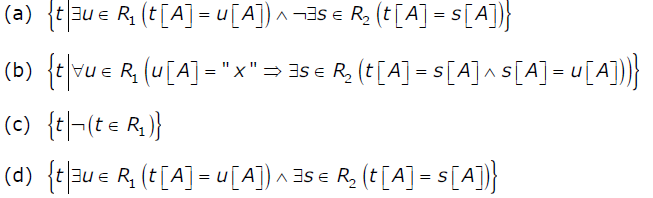# GATE | GATE-CS-2001 | Question 49

Which of the following relational calculus expressions is not safe?(A) A
(B) B
(C) C
(D) D

Explanation: A tuple relational calculus expression may at times generate an infinite relation. It may also contain values that do not even appear in the database. Such expressions are said to be unsafe.
A
safe tuple relational calculus expression is the one which surely generates finite results.
To pose a restriction over the unsafety of expressions in tuple relational calculus there is a concept of
domain of a tuple relational formula denoted by dom (P) is the set of values referenced by P i.e. values there in P or values in tuple of a relation mentioned in P.

Eg: The expression {t | ¬ (t € R)} is not safe because there are infinitely many tuples that do not occur in R relation .

In the above question Options (A), (B) and option (D) produce finite set of tuples as each gives out tuples restricted from a particular relation and hence are safe.
Option (C) produces infinite number of tuples as it generates all the tuples not in R1 i.e. it can have tuples from any other relation other than R1.Hence it is not safe.

codex.cs.yale.edu/avi/db-book/db6/slide-dir/PPT-dir/ch6.ppt

This solution is contributed by Yashika Arora.